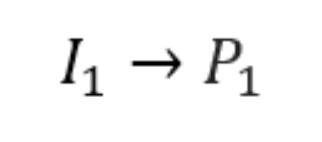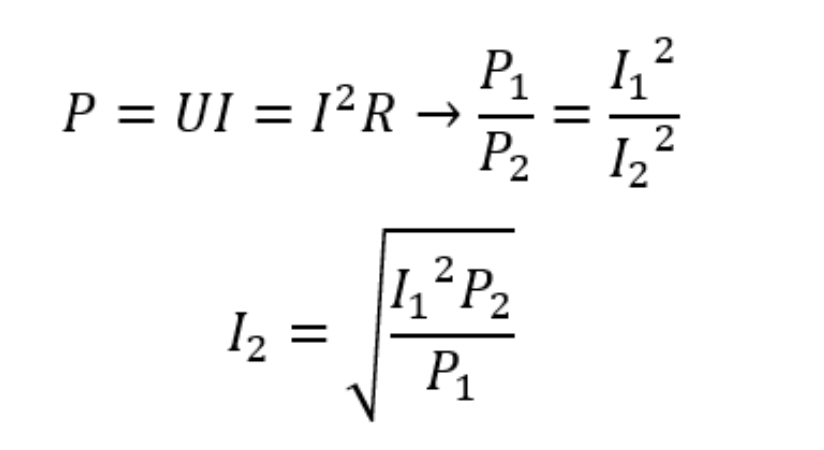top of page
Search

# Power control in simulations

Power control is the most widely used induction heating process control available. Power supply manufacturers offer different types of control such as current, voltage or even frequency control, but the power control remains the most popular one.

Process engineers who are overseeing the heating very often do not even know the actual current or voltage values in the inductor, as it is not relevant to the process supervision. When a simulation is built to optimize the heating process, many engineers stumble upon the fact that they cannot use power as an input - instead they need to define the current or voltage in the inductor. Why?

### Physics behind an induction heating simulation

The electromagnetic side of the induction heating in its base is governed by the well-known Maxwell equations, which together define the relations between the electrical charges, currents, and electric and magnetic fields. These equations and their derivations are used not only in

CENOS, but in every simulation software which can calculate electromagnetics.

The problem is that the power value which is known to the process engineers cannot be used as an input in these equations, making it fundamentally impossible to carry out power controlled induction heating simulations.

We now understand the reason why we can't use the power as an input in a simulation, but that does not resolve the problem of what to do when only the power value is known. In such a situation multiple approaches can be used depending on the simulation setup.

### Without B(H) (constant permeability)

In everyday calculations and initial design iterations, the B(H) curve will probably not be used in material definitions to save the calculation time. If you do not use a B(H) curve in your simulation, you can easily calculate the current value which corresponds to your desired power value with just one simulation.

1. Choose one random current value and run 1 time step of purely electromagnetic simulation as it will take much less time to calculate (you can easily disable thermal analysis in the Induction Heating physics). You will find the power value corresponding to the current you defined.2. Without the B(H) curve the power value is proportional to the current. We can calculate the necessary current value if we know the relation between I1 and P1.Once you have calculated the necessary current I2 to get the desired power P2, you can enable thermal analysis, enter the calculated current value, and run the simulation with a specified power.

### With B(H)

If you want to use the B(H) curve in your material definition, input current might no longer be proportional to the output power. In such a case you need to take your best guess at what the inductor current could be and run one time step of purely electromagnetic simulation. Take a look at the power value in the results and increase or decrease the current until you reach the desired power consumption.With this approach, you will need to carry out a couple of simulations to find the correct current value. The closer your first guessed current value will be to the final (real) one, the fewer iterations you will need. If the first guess is good, typically it takes no longer than 2-3 iterations. Remember, that only the electromagnetic task calculates fastly, so it shouldn’t take more than 15-30 minutes to get to the desired current value in such an iterative algorithm.

### μ(T) (heated above Curie temperature)

If the part is heated above Curie temperature, power controlled simulation is not possible, as the impedance of the system changes around and after Curie point that causes jump in current and voltage values. Because of this, to keep the same power value, there needs to be different current or voltage values before and after the Curie point (you can see how the power value changes at constant current and voltage values).In such a case current or voltage can be defined as table values depending on time, and with multiple iterations (similar to the steps with B(H)) you can try to find the setup which corresponds to the necessary power consumption. You can run the current (or voltage) estimation procedure separately under and above Curie point, then you should figure out the time moment for this transition in the additional iterative loop.

CENOS team hopes this guide will help engineers struggling with the simulation of power controlled cases. In case you still struggle, feel free to reach out to our engineering support team.

bottom of page